# 7. Time Evolution in Phase Space: Poisson Brackets and Constants of the Motion

## The Poisson Bracket

A function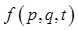of the phase space coordinates of the system and time has total time derivative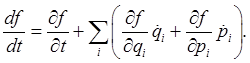This is often written as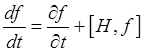whereis called the Poisson bracket. (Warning! This is Landau's definition: many others use the opposite sign.)

If, for a phase space function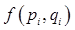(that is, no explicit time dependence)thenis a constant of the motion, also called an integral of the motion.

In fact, the Poisson bracket can be defined for any two functions defined in phase space:It's straightforward to check the following properties of the Poisson bracket: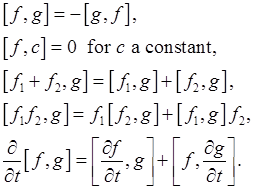The Poisson brackets of the basic variables are easily found to be: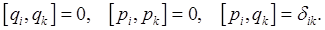Now, using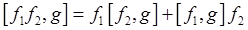and the basic variable P.B.'s,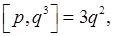and, in fact, the bracket of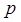with any reasonably smooth function of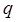is:### Interlude: a Bit of History of Quantum Mechanics

It should be clear at this point that the Poisson bracket is very closely related to the commutator in quantum mechanics. In the usual quantum mechanical notation, the momentum operator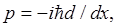so the commutator (which acts on a wave function, remember)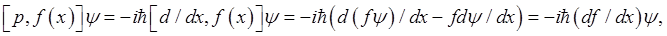identical to the Poisson bracket result multiplied by the constant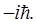The first successful mathematical formulation of quantum mechanics, in 1925 (before Schrodinger's equation!) was by Heisenberg. As you know, he was the guy with the Uncertainty Principle: he realized that you couldn't measure momentum and position of anything simultaneously. He represented the states of a quantum system as vectors in some Hilbert space, and the dynamical variables as matrices acting on these vectors. He interpreted the result of a measurement as finding an eigenvalue of a matrix. If two variables couldn't be measured at the same time, the matrices had a nonzero commutator. In particular, for a particle's position and momentum the matrix representations satisfied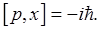Dirac made the connection with Poisson brackets on a long Sunday walk, mulling over Heisenberg's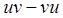(as it was written). He suddenly but dimly remembered what he called "these strange quantities" -- the Poisson brackets -- which he felt might have properties corresponding to the quantum mathematical formalism Heisenberg was building. But he didn't have access to advanced dynamics books until the college library opened the next morning, so he spent a sleepless night. First thing Monday, he read the relevant bit of Whittaker's Analytical Dynamics, and saw he was correct. (From the biography by Helge Kragh.)

Dirac went on to adapt the equationto quantum mechanics: for time-independent functions,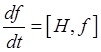, becomes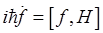for time development of an operator in the Heisenberg picture, where state vectors of closed systems do not vary in time (as opposed to the Schrodinger picture, where the vectors vary and the operators remain constant).

## The Jacobi Identity

Another important identity satisfied by the Poisson brackets is the Jacobi identity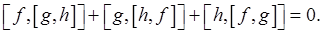This can be proved by the incredibly tedious method of just working it out. A more thoughtful proof is presented by Landau, but we're not going through it here. Ironically, the Jacobi identity is a lot easier to prove in its quantum mechanical incarnation (where the bracket just signifies the commutator of two matrix operators,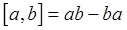).

Jacobi's identity plays an important role in general relativity.

## Poisson's Theorem

If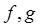are two constants of the motion (meaning they both have zero Poisson brackets with the Hamiltonian), then the Poisson bracket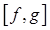is also a constant of the motion. Of course, it could be trivial, likeor it could be a function of the original variables. But sometimes it's a new constant of motion. Ifare time-independent, the proof follows immediately from Jacobi's identity. A proof for time dependent functions is given in Landau -- it's not difficult.

### Example: angular momentum components

A moving particle has angular momentum about the origin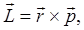soUsing the Poisson brackets found above,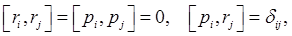we haveWe conclude that if two components of angular momentum are conserved, so is the third.

(Note: we remind the reader that we are following Landau's convention, in which the Poisson brackets have the opposite sign to the more common use, for example in Goldstein and Wikipedia.)